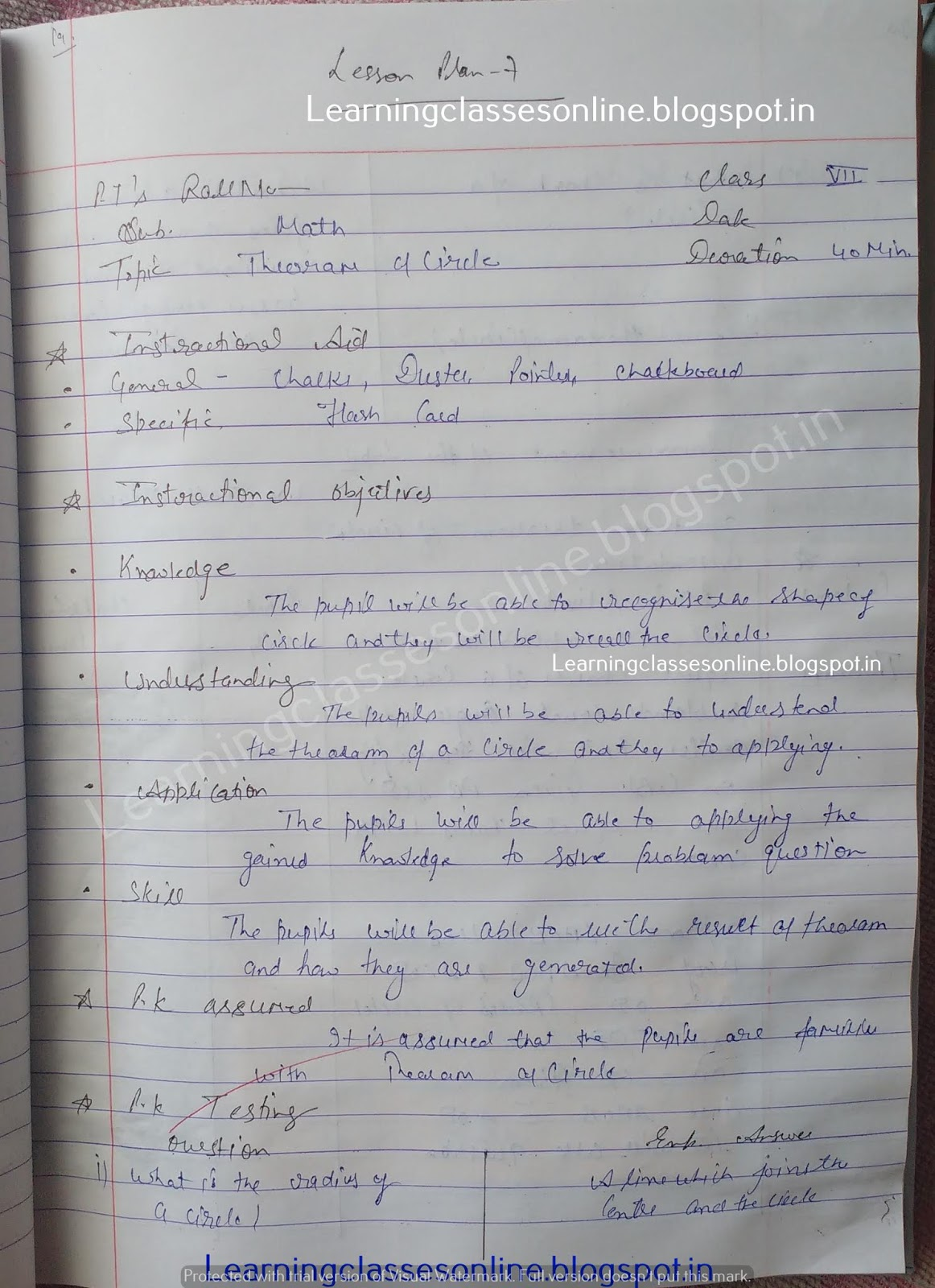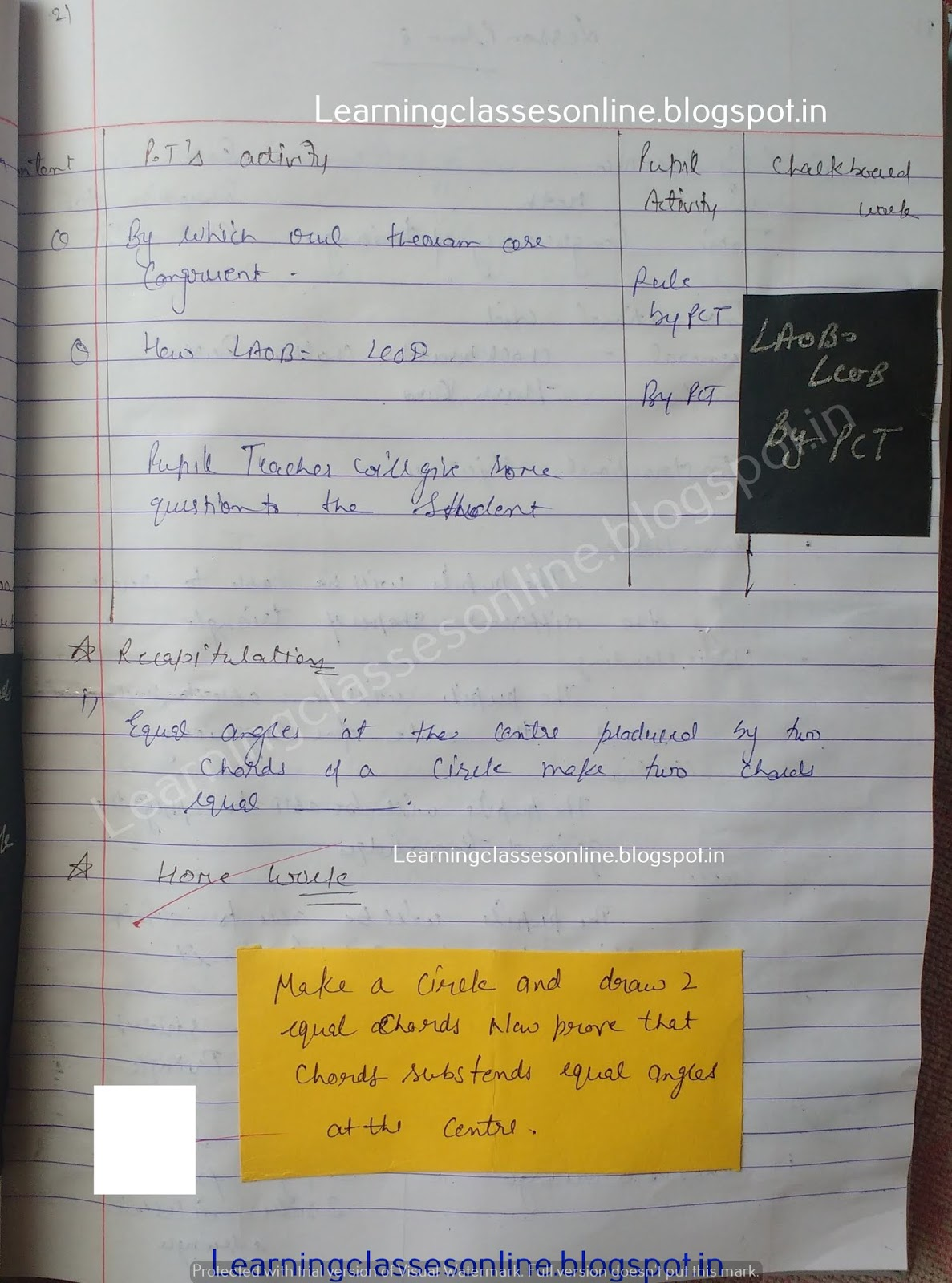Math lesson plan on theorems of circle

# Math lesson plan on theorems of circle

Math lesson plan on theorems of the circle  for class 6 , 7 , 8 and 9 teachers.

Note: The Mathematics Lesson Plan given below is just an example. You can change the Name, Class, Course, Date, Duration, etc. according to your needs.Further Reference:
www.learningclassesonline.com Mathematics Lesson Plan for B.Ed